# High School Chemistry : Using Orbital Notation

## Example Questions

### Example Question #1 : Using Orbital Notation

Choose the answer with the correct electron configuration for a sodium atom.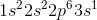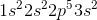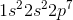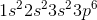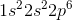Explanation:is the correct configuration for a neutral sodium atom.

A neutral sodium atom contains eleven electrons, so you can eliminateandwhich contain twelve and ten electrons, respectively.

Next,can be eliminated because of the Aufbau principal, which states that electrons are placed into orbitals from the lowest to highest energy. In this answer choice, the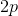(lower energy) orbital is not completely filled, containing only five out of the possible six possible electron that it can hold. This orbital must be filled completely before electrons can be placed in the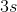(higher energy) orbital.is incorrect because there are seven electrons in theorbital. This is impossible, as p orbitals hold a maximum of six electrons.

### Example Question #31 : Elements And Compounds

How many valence electrons are in an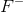ion?Explanation:

The noble gas electron configuration for fluorine,, in its ground state is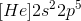. We can see from this configuration that there are seven valence electron in its outer shell of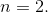When an element gains or loses charge, becoming a cation or an anion, it is either gaining or loosing electrons. Anions (negatively charged ions) have gained electrons and cations (positively charged ions) have lost electrons.

Theion has gained one electron, increasing its total valence electrons by one. The new noble gas electron configuration for the ion will be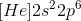, with eight valence electrons. The ion is now isoelectronic to the noble gas neon, and satisfies the octet rule.

### Example Question #32 : Elements And Compounds

Choose the correct noble gas configuration for sulfur.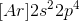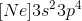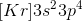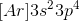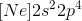Explanation:

To write the noble gas configuration of an element, choose the noble gas in the row above the element to put in brackets. For sulfur, that is the noble gas neon,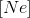.

Then, you write the rest of the configuration beginning on the same row as the given element. Sulfur is in the third row, or period, which means that it must start with theorbital. Continuing to fill the orbitals, we get the configuration.

Writing electron configuration this way can save time, and easily tells us how many valence electrons an element has. Sulfur has six valence electrons, shown by the number of electrons in the third energy level.

### Example Question #112 : High School Chemistry

A ground state atom of phosphorus will have how many valence electrons?Explanation:

In its ground state, phosphorous has five valence electron.

We can determine the number of valence electrons by examining the ground state electron configuration of an element. The ground state electron configuration of phosphorous is: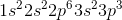From this configuration we can see that the outermost shell is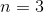. In its outer shell there are two electrons in thesubshell and three electrons in thesubshell, giving us a total of five valence electrons. Keep in mind that the different subshells correspond to different orbitals in the same energy level. When determining valence electrons, all electrons in the highest energy level must be included.

### Example Question #111 : High School Chemistry

Which of these ions does not have a noble gas electron configuration?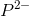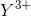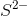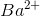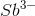All of the answer choices, except, have octet/noble gas electron configurations. Phosphorus (P), at its ground state, has 5 valence electrons. A -2 charge will create an ion with 7, not 8, electrons.